Transum search results ...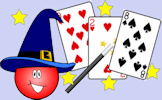Mathemagic

Mathematical tricks and illusions that anyone can understand with a little mathematical knowledge.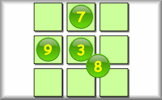Magic Square

Each row, column and diagonal should produce the same sum.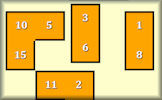Magic Square Jigsaws

Interactive jigsaw puzzles of four by four magic squares.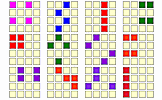Magic Square Puzzle

Find all of the possible ways of making the magic total from the numbers in this four by four magic square.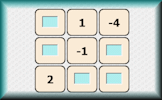Negative Magic

Four partially completed magic squares containing negative numbers. Fill in the missing numbers.Perfect Magic Square

Arrange the sixteen numbers on the four by four grid so that groups of four numbers in a pattern add up to the same total.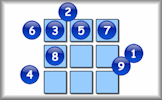Unmagic Square

Like the magic square but all of the totals should be different.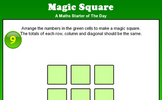Magic Square

Arrange the numbers 1 to 9 in a 3 by 3 grid to form a magic square.Unmagic Square

Arrange the numbers 1 to 9 in a 3 by 3 grid so that none of the line totals are the same.

FREE mathematical activities, puzzles, problems, visual aids, investigations and lots more.
Enter Transum Maths to see how teaching and learning this fascinating subject can be fun.You can enter the site by clicking on the apple picture above or any of the other links on this page
assuming you accept our policy.

Transum.org [also at Transum.info and Transum.com] developed as an eclectic mix of free webware featuring online activities to help you with Mathematics. You can read the latest Press Release or see the other parts of the site.